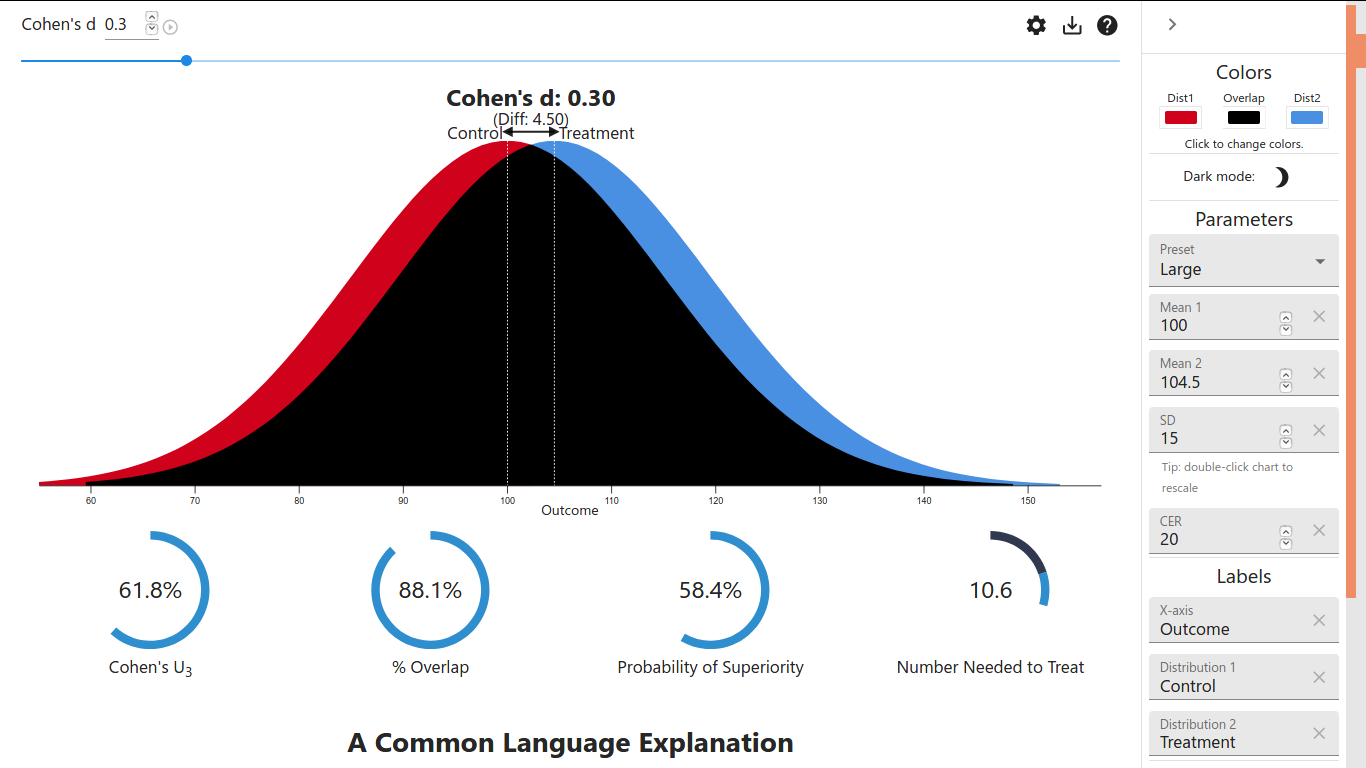# P value or Effect size

Example## Definitions¶

### Effect size¶

Note

In statistics, an effect size is a number measuring the strength of the relationship between two variables in a statistical population, or a sample-based estimate of that quantity...Examples of effect sizes include ... mean difference.

Note

In medical education research studies that compare different educational interventions, effect size is the magnitude of the difference between groups.

Note

Thus, effect size can refer to the raw difference between group means, or absolute effect size, as well as standardized measures of effect, which are calculated to transform the effect to an easily understood scale.

### Cohen's d¶

Note

Cohen's d is an effect size used to indicate the standardised difference between two means.

$s = \sqrt{\frac{(n_1-1)s_{1}^{2} + (n_2-1)s_{2}^{2}}{n_1 + n_2 - 2}}$
$s_{1}^{2} = \frac{1}{n_1-1}\sum_{i=1}^{n_1}(x_{1, i}-\bar{x}_1)^2$

## How¶

Overlap: overlapping coefficient (OVL): $$OVL = 2 \Phi(-|\delta|/2) = 2\Phi(-0.15) = 2 \times 0.4403823 = 0.8807646 \approx 88\%$$ 也就是上面例子中重叠占比88%的由来。注意这里$$\Phi$$为标准正态分布的累积密度函数(CDF), 这里的$$\Phi(-0.15)$$在R语言用pnorm(-0.15)即可算得.

Probability of superiority: common language effect size (CL): $$CL = \Phi(\frac{\delta}{\sqrt2}) = \Phi(0.3/\sqrt2) = 0.583998 \approx 58.4\%$$ 也就是上面例子中58.4%的由来。

Number Needed to Treat(NNT): $$NNT = \frac{1}{\Phi(\delta + \Psi(CER))- CER} = \frac{1}{\Phi(0.3 + \Psi(0.2))- 0.2} = 10.6338$$ 关于这里的CER参考附录CER&EER&NNT. 这里$$\Psi$$函数为$$\Phi$$的反函数，在R中为qnorm. 上述计算就是10.6的由来。也就是说，那个例子的计算中是默认了CER为0.2的...

TODO: 至于例子中这个9.4, 我直观感觉应该是$$100 \times \frac{1}{10.6}=9.433962$$，但是有点绕不过来Orz

Cohen's $$U_3$$: $$U_3 = \Phi(\delta) = \Phi(0.3) = 0.6179114 \approx 61.8\%$$

Tip

## 附录:CER&EER&NNT¶

Event rate: The proportion of patients in a group in whom the event is observed. Thus, if out of 100 patients, the event is observed in 27, the event rate is 0.27 or 27%. Control event rate (CER) and experimental event rate (EER) are used to refer to this in control and experimental groups of patients respectively. An example might help.

Example

Treatment 总人数 疼痛减少50%以上的人数 疼痛没有减少50%以上的人

Experimental event rate (EER, event rate with ibuprofen): 22/40 = 0.55 or 55%

Control event rate(CER, event rate with placebo): 7/40 = 0.18 or 18%

Absolute risk increase or reduction (EER-CER): 0.55 - 0.18 = 0.37 or 37%

NNT (1/(EER-CER)):1/(0.55 - 0.18) = 2.7